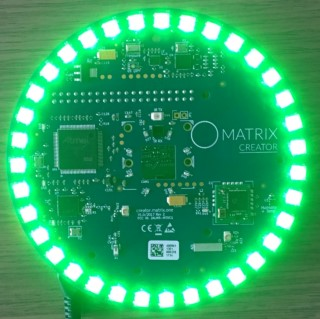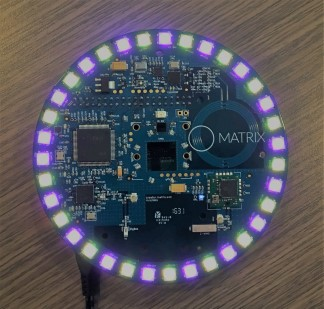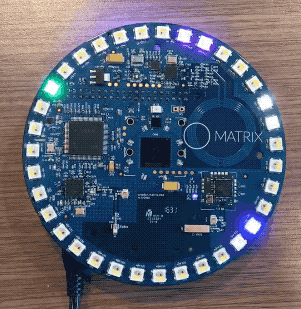# Everloop

## Everloop

### Device Compatibility## Overview

The Everloop interface supports:

• Setting the RGBW colors for each individual LED.

## Code Examples

Below are examples of how to interface with the Everloop in MATRIX HAL.

Everloop function references can be found here.

The command below will compile each example. Be sure to pass in your C++ file and desired output file.

```g++ -o YOUR_OUTPUT_FILE YOUR_CPP_FILE -std=c++11 -lmatrix_creator_hal
```
Everloop Green

The following section shows how to set the Everloop to green. You can download this example here.

#### ResultInclude Statements

To begin working with the Everloop you need to include these header files.

```// System calls
#include <unistd.h>
// Input/output streams and functions
#include <iostream>

// Interfaces with Everloop
#include "matrix_hal/everloop.h"
// Holds data for Everloop
#include "matrix_hal/everloop_image.h"
// Communicates with MATRIX device
#include "matrix_hal/matrixio_bus.h"
```
Initial Setup

You'll then need to setup `MatrixIOBus` in order to communicate with the hardware on your MATRIX device.

```int main() {
// Create MatrixIOBus object for hardware communication
matrix_hal::MatrixIOBus bus;
// Initialize bus and exit program if error occurs
if (!bus.Init()) return false;
```
Main Setup

Now we will create our `EverloopImage` and `Everloop` object and use it to set the Everloop to green for 10 seconds.

```// The following code is part of main()

// Holds the number of LEDs on MATRIX device
int ledCount = bus.MatrixLeds();
// Create EverloopImage object, with size of ledCount
matrix_hal::EverloopImage everloop_image(ledCount);
// Create Everloop object
matrix_hal::Everloop everloop;
// Set everloop to use MatrixIOBus bus
everloop.Setup(&bus);

// For each led in everloop_image.leds, set led value
for (matrix_hal::LedValue &led : everloop_image.leds) {
led.red = 0;
// Set green to 100
led.green = 100;
led.blue = 0;
led.white = 0;
}

// Updates the Everloop on the MATRIX device
everloop.Write(&everloop_image);

// Output everloop status to console
std::cout << "Everloop set to green for 10 seconds." << std::endl;

for (int i = 0; i <= 10; i++) {
// Output time remaining to console
std::cout << "Time remaining (s) : " << 10 - i << std::endl;
// Sleep for 1 second
usleep(1000000);
}

// For each led in everloop_image.leds, set led value to 0
for (matrix_hal::LedValue &led : everloop_image.leds) {
// Turn off Everloop
led.red = 0;
led.green = 0;
led.blue = 0;
led.white = 0;
}

// Updates the Everloop on the MATRIX device
everloop.Write(&everloop_image);

return 0;
}
```
Everloop Yellow/Purple

The following section shows how to set the Everloop to yellow and purple (with color blending). You can download this example here.

#### ResultInclude Statements

To begin working with the Everloop you need to include these header files.

```// System calls
#include <unistd.h>
// Input/output streams and functions
#include <iostream>

// Interfaces with Everloop
#include "matrix_hal/everloop.h"
// Holds data for Everloop
#include "matrix_hal/everloop_image.h"
// Communicates with MATRIX device
#include "matrix_hal/matrixio_bus.h"
```
Initial Setup

You'll then need to setup `MatrixIOBus` in order to communicate with the hardware on your MATRIX device.

```int main() {
// Create MatrixIOBus object for hardware communication
matrix_hal::MatrixIOBus bus;
// Initialize bus and exit program if error occurs
if (!bus.Init()) return false;
```
Main Setup

Now we will create our `EverloopImage` and `Everloop` object and use it to set the Everloop to yellow and purple for 10 seconds.

```// The following code is part of main()

// Holds the number of LEDs on MATRIX device
int ledCount = bus.MatrixLeds();
// Create EverloopImage object, with size of ledCount
matrix_hal::EverloopImage everloop_image(ledCount);
// Create Everloop object
matrix_hal::Everloop everloop;
// Set everloop to use MatrixIOBus bus
everloop.Setup(&bus);

// For each led in everloop_image.leds, set led value
for (int i = 0; i < everloop_image.leds.size(); i++) {
// When remainder is zero set led to purple
if (i % 2 == 0) {
// Blending blue and red to make purple
everloop_image.leds[i].blue = 40;
everloop_image.leds[i].red = 40;
}
// Else set led to yellow
else {
// Blending green and red to make yellow
everloop_image.leds[i].green = 40;
everloop_image.leds[i].red = 60;
}
}

// Updates the Everloop on the MATRIX device
everloop.Write(&everloop_image);

// Output everloop status to console
std::cout << "Everloop set to yellow and purple for 10 seconds." << std::endl;

for (int i = 0; i <= 10; i++) {
// Output time remaining to console
std::cout << "Time remaining (s) : " << 10 - i << std::endl;
// Sleep for 1 second
usleep(1000000);
}

// For each led in everloop_image.leds, set led value to 0
for (matrix_hal::LedValue &led : everloop_image.leds) {
// Turn off Everloop
led.red = 0;
led.green = 0;
led.blue = 0;
led.white = 0;
}

// Updates the Everloop on the MATRIX device
everloop.Write(&everloop_image);

return 0;
}
```
Everloop Rainbow

The following section shows how to set the Everloop to rainbow. You can download this example here.

#### ResultInclude Statements

To begin working with the Everloop you need to include these header files.

```// System calls
#include <unistd.h>
// Input/output streams and functions
#include <iostream>
// Included for sin() function.
#include <cmath>

// Interfaces with Everloop
#include "matrix_hal/everloop.h"
// Holds data for Everloop
#include "matrix_hal/everloop_image.h"
// Communicates with MATRIX device
#include "matrix_hal/matrixio_bus.h"
```
Initial Setup

You'll then need to setup `MatrixIOBus` in order to communicate with the hardware on your MATRIX device.

```int main() {
// Create MatrixIOBus object for hardware communication
matrix_hal::MatrixIOBus bus;
// Initialize bus and exit program if error occurs
if (!bus.Init()) return false;
```
Main Setup

Now we will create our `EverloopImage` and `Everloop` object and use it to set the Everloop to rainbow for 10 seconds.

```// The following code is part of main()

// Holds the number of LEDs on MATRIX device
int ledCount = bus.MatrixLeds();
// Create EverloopImage object, with size of ledCount
matrix_hal::EverloopImage everloop_image(ledCount);
// Create Everloop object
matrix_hal::Everloop everloop;
// Set everloop to use MatrixIOBus bus
everloop.Setup(&bus);

// Variables used for sine wave rainbow logic
float counter = 0;
const float freq = 0.375;

// 10 sec loop for rainbow effect 250*40000 microsec = 10 sec
for (int i = 0; i <= 250; i++) {
// For each led in everloop_image.leds, set led value
for (matrix_hal::LedValue &led : everloop_image.leds) {
// Sine waves 120 degrees out of phase for rainbow
led.red =
(std::sin(freq * counter + (M_PI / 180 * 240)) * 155 + 100) / 10;
led.green =
(std::sin(freq * counter + (M_PI / 180 * 120)) * 155 + 100) / 10;
led.blue = (std::sin(freq * counter + 0) * 155 + 100) / 10;
// If MATRIX Creator, increment by 0.51
if (ledCount == 35) {
counter = counter + 0.51;
}
// If MATRIX Voice, increment by 1.01
if (ledCount == 18) {
counter = counter + 1.01;
}
}

everloop.Write(&everloop_image);

// If i is 0 (first run)
if (i == 0) {
// Output everloop status to console
std::cout << "Everloop set to rainbow for 10 seconds." << std::endl;
}
// If i is cleanly divisible by 25
if ((i % 25) == 0) {
std::cout << "Time remaining (s) : " << 10 - (i / 25) << std::endl;
}

// Sleep for 40000 microseconds
usleep(40000);
}

// Updates the Everloop on the MATRIX device
everloop.Write(&everloop_image);

// For each led in everloop_image.leds, set led value to 0
for (matrix_hal::LedValue &led : everloop_image.leds) {
// Turn off Everloop
led.red = 0;
led.green = 0;
led.blue = 0;
led.white = 0;
}

// Updates the Everloop on the MATRIX device
everloop.Write(&everloop_image);

return 0;
}
```
Everloop Moving Dots

The following section shows how to set the Everloop to moving dots. You can download this example here.

#### ResultInclude Statements

To begin working with the Everloop you need to include these header files.

```// System calls
#include <unistd.h>
// Input/output streams and functions
#include <iostream>
// Included for sin() function.
#include <cmath>

// Interfaces with Everloop
#include "matrix_hal/everloop.h"
// Holds data for Everloop
#include "matrix_hal/everloop_image.h"
// Communicates with MATRIX device
#include "matrix_hal/matrixio_bus.h"
```
Initial Setup

You'll then need to setup `MatrixIOBus` in order to communicate with the hardware on your MATRIX device.

```int main() {
// Create MatrixIOBus object for hardware communication
matrix_hal::MatrixIOBus bus;
// Initialize bus and exit program if error occurs
if (!bus.Init()) return false;
```
Main Setup

Now we will create our `EverloopImage` and `Everloop` object and use it to set the Everloop to moving dots for 10 seconds.

```// The following code is part of main()

// Holds the number of LEDs on MATRIX device
int ledCount = bus.MatrixLeds();
// Create EverloopImage object, with size of ledCount
matrix_hal::EverloopImage everloop_image(ledCount);
// Create Everloop object
matrix_hal::Everloop everloop;
// Set everloop to use MatrixIOBus bus
everloop.Setup(&bus);

// Keeps track of location of moving dots
long counter = 0;

// 10 sec loop for rainbow effect 500*20000 microsec = 10 sec
for (int i = 0; i <= 500; i++) {
// For each led in everloop_image.leds, set led value to 0
for (matrix_hal::LedValue &led : everloop_image.leds) {
// Turn off Everloop
led.red = 0;
led.green = 0;
led.blue = 0;
led.white = 0;
}

// Set led color per led
everloop_image.leds[(counter / 2) % everloop_image.leds.size()].red = 40;
everloop_image.leds[(counter / 2) % everloop_image.leds.size()].blue = 40;
everloop_image.leds[(counter / 7) % everloop_image.leds.size()].green = 60;
everloop_image.leds[(counter / 11) % everloop_image.leds.size()].blue = 60;
everloop_image
.leds[everloop_image.leds.size() - 1 -
(counter % everloop_image.leds.size())]
.white = 20;

// Updates the Everloop on the MATRIX device
everloop.Write(&everloop_image);
// Increment counter
counter++;

// If i is 0 (first run)
if (i == 0) {
// Output everloop status to console
std::cout << "Everloop set to moving dots for 10 seconds." << std::endl;
}
// If i is cleanly divisible by 50
if ((i % 50) == 0) {
// Output time remaining to console
std::cout << "Time remaining (s) : " << 10 - (i / 50) << std::endl;
}

// Sleep for 20000 microseconds
usleep(20000);
}

// Updates the Everloop on the MATRIX device
everloop.Write(&everloop_image);

// For each led in everloop_image.leds, set led value to 0
for (matrix_hal::LedValue &led : everloop_image.leds) {
// Turn off Everloop
led.red = 0;
led.green = 0;
led.blue = 0;
led.white = 0;
}

// Updates the Everloop on the MATRIX device
everloop.Write(&everloop_image);

return 0;
}
```